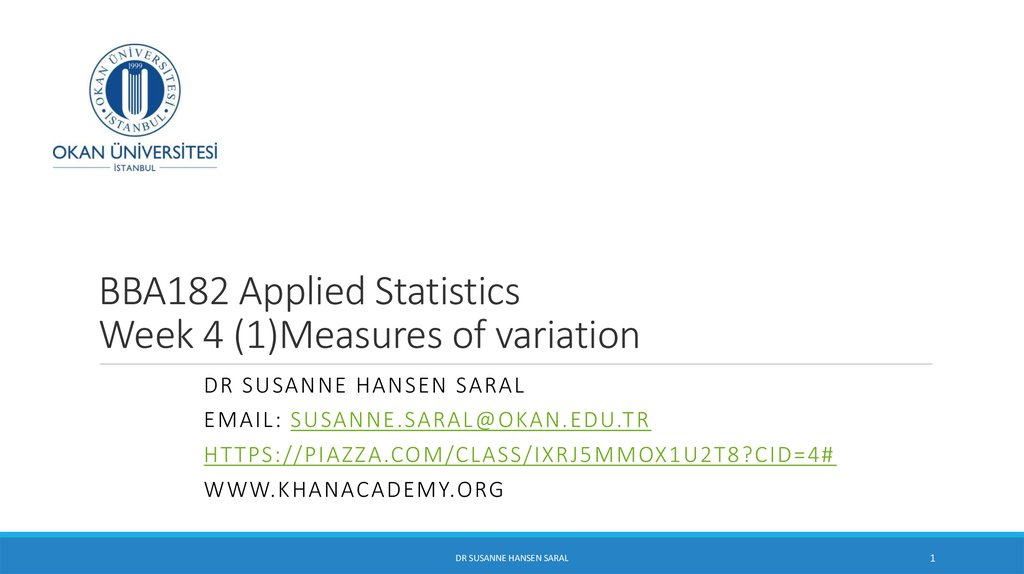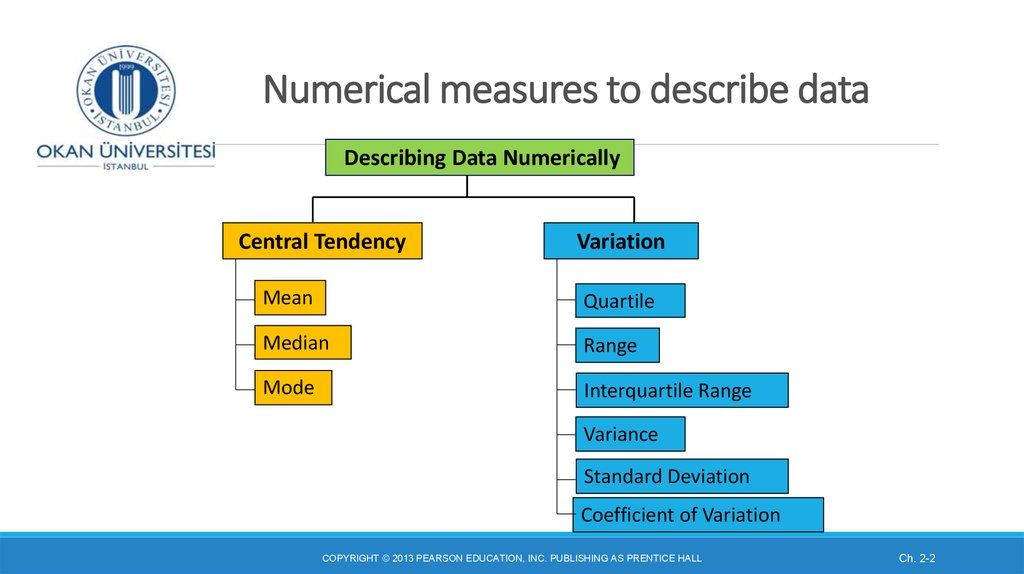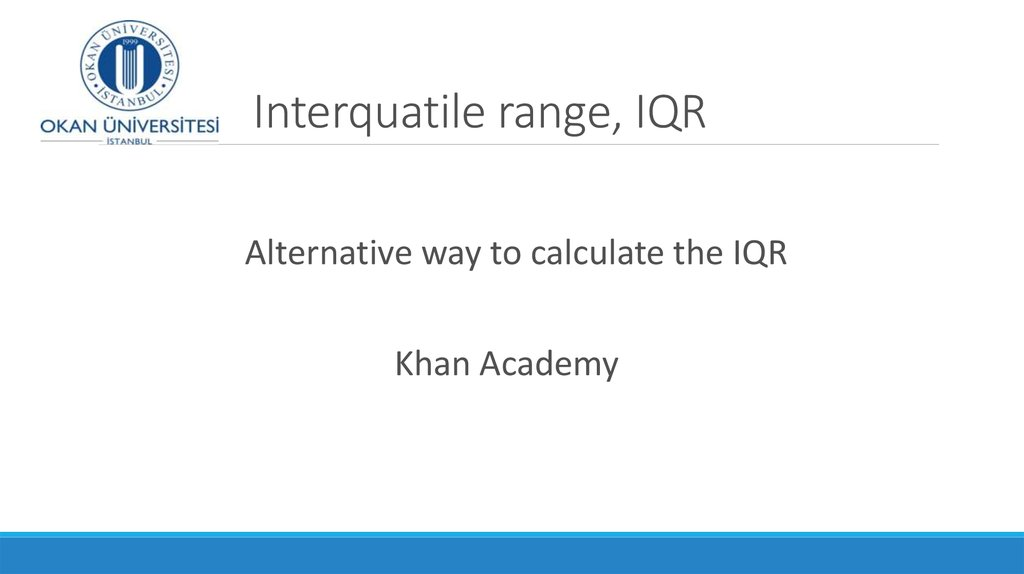# Measures of variation. Week 4 (1)

## 1. BBA182 Applied Statistics Week 4 (1)Measures of variation

DR SUSANNE HANSEN SARAL
EMAIL: [email protected]
HT TPS://PIAZZA.COM/CLASS/IXRJ5MMOX1U2T8?CID=4#
DR SUSANNE HANSEN SARAL
1

## 2. Numerical measures to describe data

Describing Data Numerically
Central Tendency
Variation
Mean
Quartile
Median
Range
Mode
Interquartile Range
Variance
Standard Deviation
Coefficient of Variation
Ch. 2-2

## 3. Interquatile range, IQR

Alternative way to calculate the IQR

## 5. Five-Number Summary of a data set

In describing numerical data, statisticians often refer to the
five-number summary. It refers to five the descriptive
measures we have looked at:
minimum value
first quartile
median
third quartile
maximum value
minimum < Q1 < median < Q3 < maximum
It gives us a good idea where the data is located and how it is spread in the
data set
DR SUSANNE HANSEN SARAL

## 6. Five-Number Summary: Example

Sample Ranked Data:
minimum <
6
6 7 8 9 10 11
Q1 < median <
< 7.75 < 10.5
Q3
11 12
< maximum
< 12.25 <
DR SUSANNE HANSEN SARAL
13 14
14

## 7. Exercise

Consider the data given below:
110
a.
b.
c.
d.
e.
f.
g.
125
99
115
119 95 110
Compute the mean.
Compute the median.
What is the mode?
What is the shape of the distribution?
What is the lower quartile, Q1?
What is the upper quartile, Q3?
Indicate the five number summary
132
85

## 8. Exercise

Consider the data given below.
85
95
99
110
110
115
119
125
132
a.
Compute the mean. 110
b.
Compute the median. 110
c.
What is the mode? 110
d.
What is the shape of the distribution? Symmetric, because mean = median=mode
e.
What is the lower quartile, Q1? 97
f.
What is the upper quartile, Q3? 122
g.
Indicate the five number summary 85 < 97 < 110 < 122 < 132

## 9. Five number summary and Boxplots

Boxplot is created from the five-number summary
A boxplot is a graph for numerical data that describes the shape of
a distribution, in terms of the 5 number summary.
It visualizes the spread of the data in the data set.
Ch. 2-9

## 10. Five number summary and Boxplots

Boxplot is created from the five-number summary
The central box shows the middle half of the data from Q1 to Q3,
(middle 50% of the data) with a line drawn at the median
Two lines extend from the box. One line is the line from Q1 to the
minimum value, the other is the line from Q3 to the maximum value
A boxplot is a graph for numerical data that describes the shape of a
distribution, like the histogram